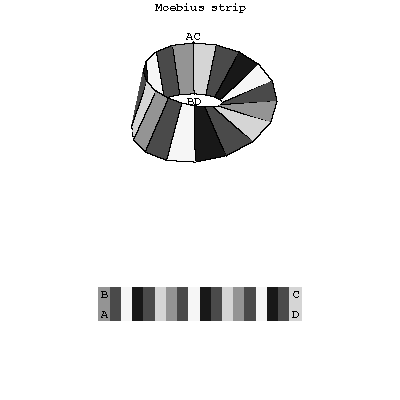# Difference between revisions of "Möbius strip"

A non-orientable surface with Euler characteristic zero whose boundary is a closed curve. The Möbius strip can be obtained by identifying two opposite sides \$AB\$ and \$CD\$ of a rectangle \$ABCD\$ so that the points \$A\$ and \$B\$ are matched with the points \$C\$ and \$D\$, respectively (see Fig.).Figure: m064310a

In the Euclidean space \$E^3\$ the Möbius strip is a one-sided surface (see One-sided and two-sided surfaces).

The Möbius strip was considered (in 1858–1865) independently by A. Möbius and I. Listing.

How to Cite This Entry:
Möbius strip. Encyclopedia of Mathematics. URL: http://encyclopediaofmath.org/index.php?title=M%C3%B6bius_strip&oldid=22819
This article was adapted from an original article by A.B. Ivanov (originator), which appeared in Encyclopedia of Mathematics - ISBN 1402006098. See original article